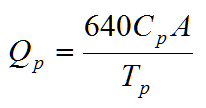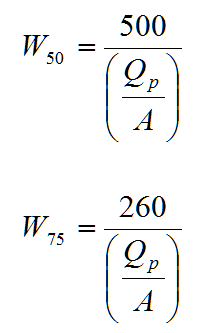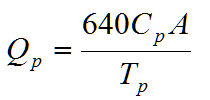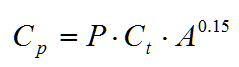#INFOSEWER

# The Colorado Urban Hydrograph Procedure in InfoSewer

The Colorado Urban Hydrograph Procedure in InfoSewer

The Colorado Urban Hydrograph Procedure (CUHP) uses the equations and procedures presented in the Urban Drainage Criteria Manual (USDCM) of the Urban Drainage and Flood Control District (UDFCD). Shape of the CUHP synthetic unit hydrograph is determined using the following equations that relate unit hydrograph parameters to catchment properties.Lag time (tl) of the watershed (catchment), defined as the time from the center of unit storm duration to the peak of the unit hydrograph, is determined as:where tl = lag time in hours.

L = length along the drainageway path from study point to the most upstream limits of the catchment in miles.

Lca = length along stream from study point to apoint along stream adjacent to the centroid of the catchment in miles.

S = length weighted average slope of catchment along draiangeway path to upstream limits of the catchment.

Ct = time to peak coeficient.

Once the lag time is known, time to peak (Tp) of the unit hydrograph could be determined by adding 0.5tr to the lag time in consistent units.

Peak flow rate, Qp, of the unit hydrograph is calculated as:where Qp = peak flow rate of the unit hydrograph, in cfs.

A = area of the catchment, in square miles.

Cp = unit hydrograph peaking coefficient, and is determined as:where P = peaking parameter.

Ct and P are defined in terms of percent impervious (Ia) of the catchment as:The coefficients a, b, c, d, e, and f are defined in terms of Ia in the following table.The widths of the unit hydrograph at 50% and 75% of the peak are estimated as:where W50 = width of the unit hydrograph at 50% of the peak, in hours.

W75 = width of the unit hydrograph at 75% of the peak, in hours.

Qp = peak flow rate, in cfs.

A = catchment area, in square miles.

It is recommended that a unit hydrograph duration of 5-minute be used for studies that apply the CUHP. The maximum recommended drainage area (catchment size) for any single CUHP unit hydrograph is 5 square miles. Whenever a larger watershed is studied, it needs to be subdivided into subcatchments of 5-square miles or less. For this synthetic unit hydrograph method, the minimum drainage area should be 90 acres. For catchments smaller than 90 acres, other unit hydrograph generation mechanisms should be used.

The Colorado Urban Hydrograph Procedure from Innovyze H2OCalc for Reference

The Colorado Urban Hydrograph Procedure (CUHP) uses the equations and procedures presented in the Urban Drainage Criteria Manual (USDCM) of the Urban Drainage and Flood Control District (UDFCD 2001). Shape of the CUHP synthetic unit hydrograph is determined using the following equations that relate unit hydrograph parameters to catchment properties.

Lag time (tl) of the watershed (catchment), defined as the time from the center of unit storm duration to the peak of the unit hydrograph, is determined as:where tl = lag time in hours.

L = length along the drainageway path from study point to the most upstream limits of

the catchment in miles.

Lca = length along stream from study point to a point along stream adjacent to the centroid

of the catchment in miles.

S = length weighted average slope of catchment along drainageway path to upstream

limits of the catchment.

Ct = time to peak coefficient.Once the lag time is known, time to peak (Tp) of the unit hydrograph could be determined by adding 0.5tr to the lag time in consistent units.

Peak flow rate, Qp, of the unit hydrograph is calculated as:where Qp = peak flow rate of the unit hydrograph, in cfs.

A = area of the catchment, in square miles.

Cp = unit hydrograph peaking coefficient, and is determined as:where P = peaking parameter.

Ct and P are defined in terms of percent impervious (Ia) of the catchment as:The coefficients a, b, c, d, e, and f are defined in terms of Ia in the following table.

 IA A B C D E F Ia ≤ 10 0.0 -0.00371 0.163 0.00245 -0.012 2.16 10 < Ia ≤ 40 0.000023 -0.00224 0.146 0.00245 -0.012 2.16 Ia > 40 0.0000033 -0.000801 0.120 -0.00091 0.228 -2.06

The widths of the unit hydrograph at 50% and 75% of the peak are estimated as:where W50 = width of the unit hydrograph at 50% of the peak, in hours.

W75 = width of the unit hydrograph at 75% of the peak, in hours.

Qp = peak flow rate, in cfs.

A = catchment area, in square miles.

It is recommended that a unit hydrograph duration of 5-minute be used for studies that apply the CUHP. The maximum recommended drainage area (catchment size) for any single CUHP unit hydrograph is 5 square miles. Whenever a larger watershed is studied, it needs to be subdivided into Subcatchments of 5-square miles or less. For this synthetic unit hydrograph method, the minimum drainage area should be 90 acres. For catchments smaller than 90 acres, other unit hydrograph generation mechanisms should be used.